# Strongly Index-Guided Lasers

This is a continuation from the previous tutorial - weakly index-guided lasers.

The lateral mode control in injection lasers can be achieved using index guiding alone the junction plane. The mode control is necessary for improving the light-current linearity and the modulation response of injection lasers.

In strongly index-guided lasers, the active region is buried in higher band-gap layers (e.g., InP) on all sides. For this reason, these lasers are called buried-heterostructure lasers.

The lateral index step along the junction plane is ~ 0.2 in these laser structures and is about two orders of magnitude larger than the carrier-induced effects. As a result, the lasing characteristics of buried-heterostructure lasers are primarily determined by the rectangular waveguide that confines the mode inside the buried active region.

Lateral and transverse modes of buried-heterostructure lasers were discussed in the waveguide modes in semiconductor lasers tutorial.

The buried heterostructure was first proposed in 1974 for GaAs lasers. Since then, several types of InGaAsP buried-heterostructure lasers have been developed. These lasers can be divided into two categories: (i) planar-active-layer structures and (ii) nonplanar-active-layer structures.

The planar-active-layer structure is more suitable for single-frequency distributed-feedback-type lasers because the waveguide modes of a planar active layer are more strongly coupled to a grating than are the waveguide modes of a nonplanar active region.

Generally, an optimized buried-heterostructure laser is characterized by a low threshold current (10-15 mA), can be operated to high output powers (~ 10 mW) in the fundamental transverse mode, and can be modulated at high frequencies (~ 2 GHz).

Buried-heterostructure lasers are more difficult to fabricate than weakly index-guided or gain-guided lasers. However, these strongly index-guided structures are most suitable for demanding applications. In particular, they are useful as a source in high-bit-rate long-haul lightwave transmission systems.

Figure 5-18 shows a schematic cross section of several different types of buried-heterostructure lasers with a planar active layer. They are

• (i) The buried heterostructure that we shall call the etched-mesa buried heterostructure (EMBH) in order to distinguish it from other buried-heterostructure lasers
• (ii) The double-channel planar buried heterostructure (DCPBH)
• (ii) The planar buried heterostructure
• (iv) The strip buried heterostructure

Variations of these structures also exist in the literature.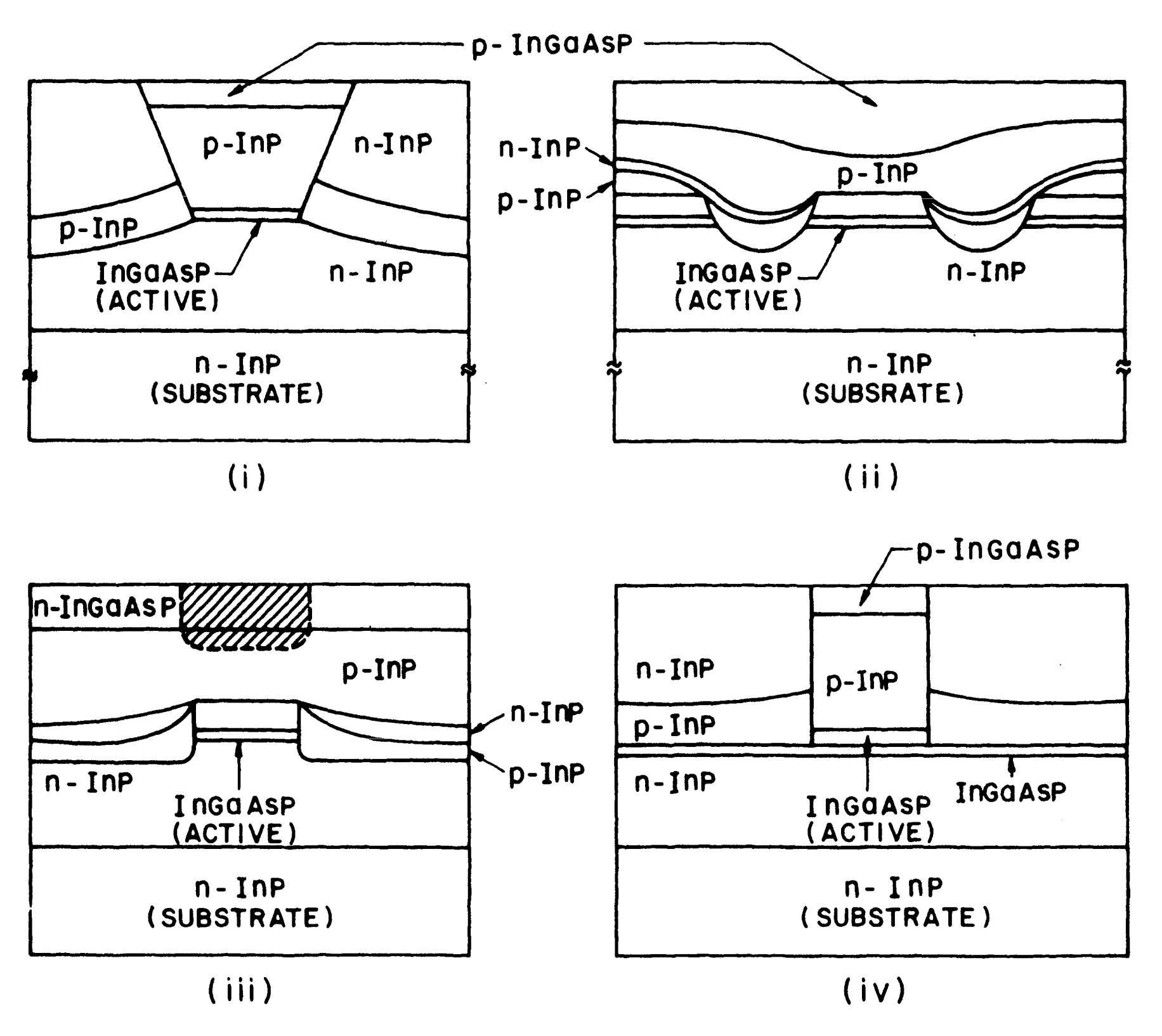Figure 5-18. Schematic cross section of different types of buried-heterostructure lasers with a planar active layer: (i) etched-mesa buried heterostructure; (ii) double-channel planar buried heterostructure; (iii) planar buried heterostructure, and (iv) strip buried heterostructure.

Figure 5-19 shows the schematic cross section of several nonplanar-active-layer laser structures. They are

(i) The V-grooved substrate buried-heterostructure laser, also known as the channeled-substrate buried-heterostructure (CSBH) laser or the buried crescent laser

(ii) The mesa-substrate buried-heterostructure (MSBH) laser

(iii) The buried-crescent buried-heterostructure (BCBH) laser

Variations of these laser structures utilizing different current-confining schemes have also been proposed.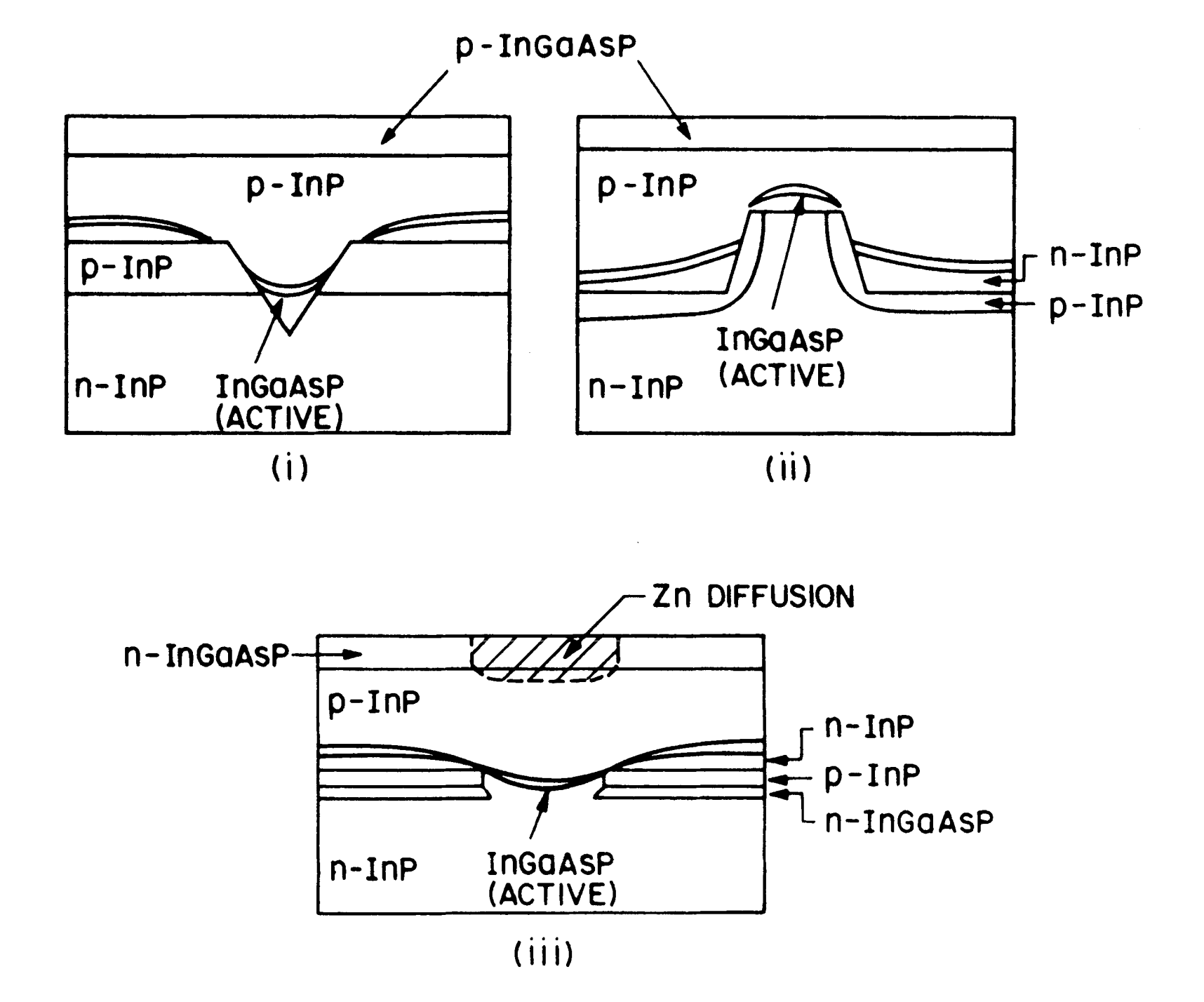Figure 5-19.  Schematic cross section of different buried-heterostructure lasers with a nonplanar active layer: (i) V-grooved substrate or channeled-substrate buried heterostructure; (ii) mesa-substrate buried heterostructure; and (iii) buried crescent.

We now describe the fabrication and performance characteristics of some of these laser structures.

Etched-Mesa Buried-Heterostructure (EMBH) Laser

Figure 5-20 shows an SEM photomicrograph of an EMBH laser. This device structure was first reported by Hirao et al.

The fabrication of this structure involves two epitaxial growths.

In the first, four layers are grown (by LPE, VPE, or MOVPE) on a (100)-oriented n-InP substrate. The four epitaxial layers are:

• (i) n-InP buffer layer ($$\sim2\times10^{18}\text{ cm}^{-3}$$ doping level, 3-4 μm thick);
• (ii) undoped InGaAsP active layer ($$\lambda=1.3$$ μm, 0.2 μm thick);
• (iii) p-InP cladding layer ($$\sim10^{18}\text{ cm}^{-3}$$ doping level, 2 μm thick);
• (iv) p-InGaAsP contact layer ($$\lambda\approx1.1$$ μm, $$\sim10^{19}\text{ cm}^{-3}$$ doping level, 0.5 μm thick).

Mesas 2-3 μm wide are then etched on the as-grown wafer using a solution of 1% $$\text{Br}_2$$ and $$\text{CH}_3\text{OH}$$ with plasma-deposited $$\text{SiO}_2$$ acting as the etching mask.

The etching mask is then removed and the p-InP and n-InP blocking layers are grown using a second LPE growth.

The wafer is then cleaved to produce laser diodes after p and n contact metallization.

Figure 5-21 shows the $$\text{L}-\text{I}$$ characteristics of an InGaAsP ($$\lambda=1.3$$ μm) EMBH laser at different temperatures.

These lasers typically have room-temperature threshold currents in the range of 10-15 mA and can be operated in the fundamental transverse mode to powers greater than 10 mW/facet.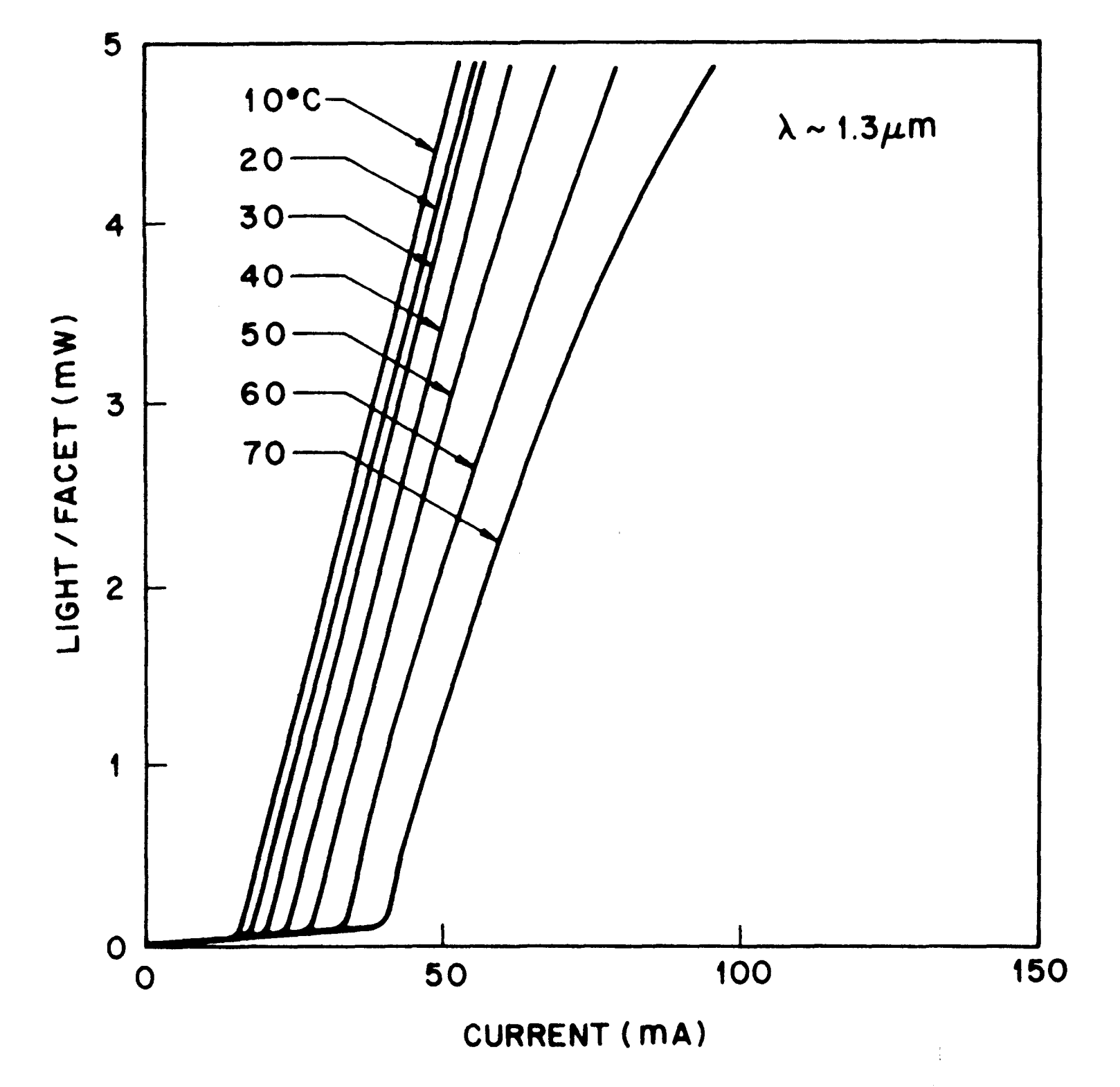Figure 5-21.  $$\text{L}-\text{I}$$ characteristics of an EMBH laser at different temperatures.

The lateral mode control in strongly index-guided lasers such as the EMBH laser can be achieved by controlling the width of the active region.

As discussed in the waveguide modes in semiconductor lasers tutorial, lasers with a wide active region allow higher-order lateral modes. Hence, with increasing current, such lasers may switch from one lateral mode to another or a higher-order lateral mode can appear in addition to the fundamental mode.

Such mode transitions are associated with a kink in the light-current characteristics similar to that shown in Figure 5-7 for a gain-guided laser [refer to the gain-guided lasers tutorial].

Figure 5-22 shows the $$\text{L}-\text{I}$$ characteristic at room temperature of an EMBH laser that exhibits a kink.

The inset in Figure 5-22 shows the measured far-field intensity along the junction plane at currents above and below the kink. The appearance of side lobes in the far field provides clear evidence that a higher-order lateral mode is lasing together with the fundamental mode.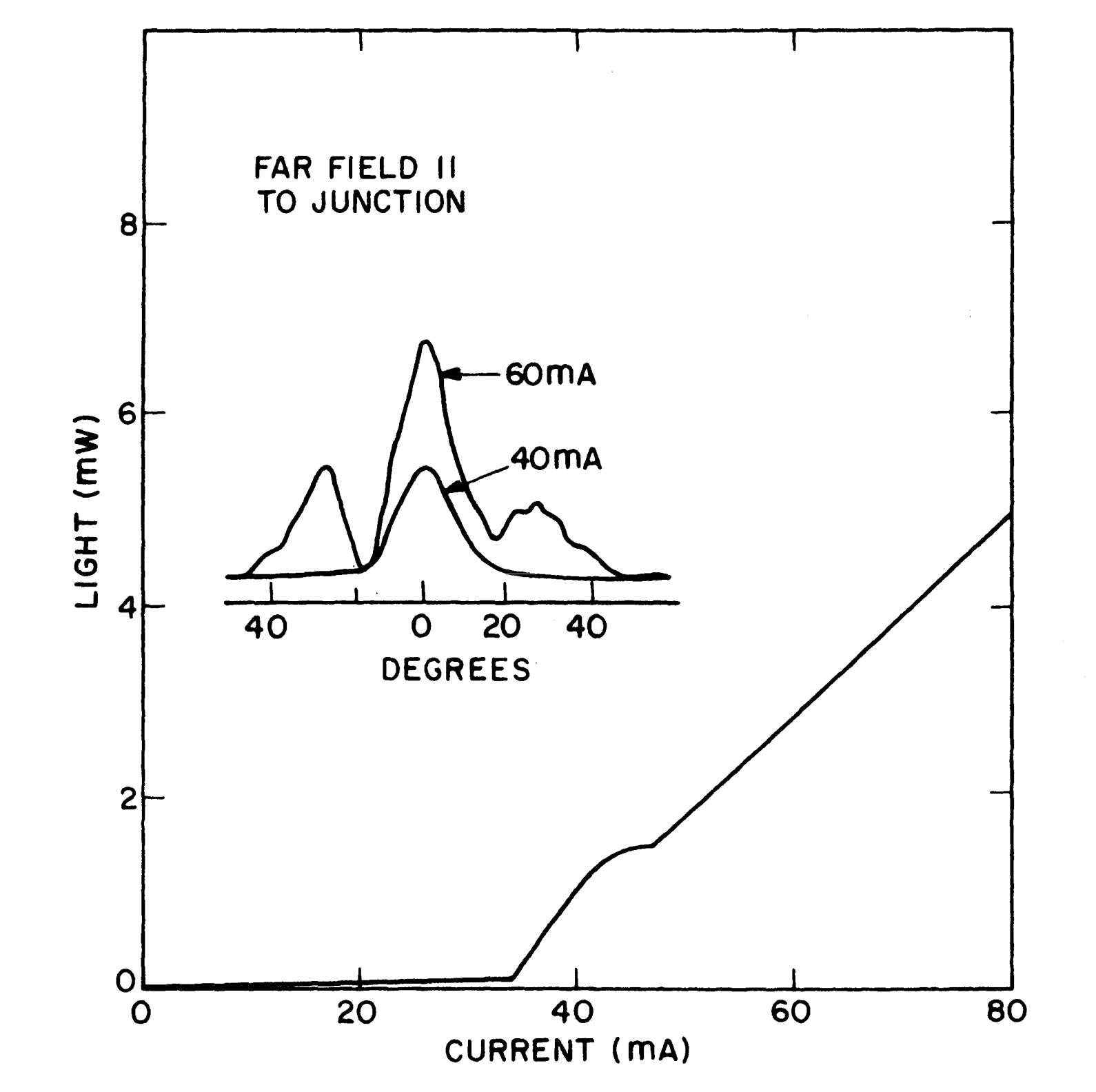Figure 5-22. $$\text{L}-\text{I}$$ characteristics of an EMBH laser showing a kink. The measured far-field along the junction plane at currents above and below the kink are also shown.

The output power at which a kink appears is called the kink power. In Figure 5-22 the kink power is about 1.5 mW. We expect the kink power to increase as the width of the active region decreases because the higher-order modes are then below or close to the cut-off level.

Figure 5-23 shows the measured kink power as a function of the active-region width of several EMBH lasers. The active-region thickness of these lasers was 0.2 μm.

The dashed curve in Figure 5-23 is drawn as a visual aid indicating that for kink powers greater than 10 mW, the active-region width must be less than 1.6 μm for a 0.2-μm-thick active layer.Figure 5-23.  Measured kink power (light output at which a kink appears) as a function of the active-region width of EMBH lasers. Arrows indicate that the kink power was more than 11 mW for these lasers. Dashed line is drawn as a visual aid.

In an EMBH laser structure, the p-n-p-n and other current-blocking junctions (see Figure 5-20) confine the current to the active region.

This current confinement is generally not complete, and a fraction of the injection current flows (or leaks) around the active region in a buried-heterostructure laser. This leakage current should be small in an optimized device structure.

An excessive leakage current can significantly affect the device threshold, the temperature performance, the modulation characteristics, and the output power characteristics.

The dependence of leakage current on various device parameters and its effect on the performance characteristics are described in detail in later part of this tutorial.

Double-Channel Planar Buried-Heterostructure (DCPBH) Lasers

Figure 5-24 shows an SEM photomicrograph of a DCPBH laser.

The active region in this device consists of a 1.3-μm InGaAsP layer.

The device fabrication involves two epitaxial growths.

In the first, four layers are grown by LPE in a manner similar to that for the EMBH laser. The top InGaAsP layer is removed using an etching mixture of $$\text{H}_2\text{SO}_4$$, $$\text{H}_2\text{O}_2$$, and $$\text{H}_2\text{O}$$ in proportions of 10:1:1.

Two channels, about 3 μm deep and 5 μm wide, are then etched on the wafer using plasma-deposited $$\text{SiO}_2$$ as the etching mask.

The mask is then removed and four epitaxial layers - p-InP, n-InP, p-InP, and p-InGaAsP ($$\lambda=1.3$$ μm) - are grown on the wafer using a second LPE growth. The last quaternary layer serves as a contact layer.

The wafer is then processed to produce laser chips in a procedure similar to that for gain-guided lasers except that the contact stripe is aligned over the active region using photolithographic techniques.

Figure 5-25 shows the light-current characteristics of a 1.3-μm InGaAsP DCPBH laser at different temperatures. The device can operate CW up to $$130^\circ\text{C}$$. DCPBH lasers typically exhibit room-temperature threshold currents in the range of 10-15 mA.

The lateral mode control is achieved by reducing the dimension of the active region. Experimental data for the kink power as a function of the active region width are similar to those shown in Figure 5-23.Figure 5-25.  $$\text{L}-\text{I}$$ characteristics of a 1.3-μm InGaAsP DCPBH laser at different temperatures.

InGaAsP lasers emitting near 1.55 μm are also of considerable interest as a source in optical fiber communication systems. The fabrication procedure for these lasers is similar to that described above except that the active-layer composition is chosen so that the band gap corresponds to the 1.55-μm wavelength.

Figure 5-26 shows the light-current characteristics at different temperatures of a DCPBH laser emitting near 1.55 μm. The laser operates CW up to $$110^\circ\text{C}$$.

A comparison of Figures 5-25 and 5-26 shows that 1.55-μm InGaAsP lasers have a higher threshold current and a lower maximum operating temperature than do 1.3-μm lasers. As discussed in the previous tutorials, increased Auger recombination at longer wavelengths is partly responsible for this behavior.

The lasers emitting near 1.55 μm also exhibit a lower differential quantum efficiency than do lasers emitting near 1.3 μm. This may be due to increased intervalence band absorption at longer wavelengths.Figure 5-26.  $$\text{L}-\text{I}$$ characteristics of a 1.55-μm InGaAsP DCPBH laser at different temperatures.

V-Grooved or Channeled-Substrate Buried-Heterostructure (CSBH) Laser

Figure 5-27 shows an SEM photomicrograph of a CSBH laser. This device structure differs from the EMBH and the DCPBH in that the active region is nonplanar.

The p-InP current-blocking layer (bottom p-InP layer in Figure 5-27) can be grown by LPE or VPE on a (100)-oriented n-InP substrate.

Sealed-tube Cd-diffusion using a $$\text{Cd}_3\text{P}_2$$ source at $$600-650^\circ\text{C}$$ can also be used to type-convert a thin layer (~ 2 μm) on the surface of n-InP substrate.

This latter method allows the fabrication of the device using a single LPE step.

V-shaped grooves are then etched on the wafer parallel to (110) using a 3:1 mixture of $$\text{HCl}$$ and $$\text{H}_3\text{PO}_4$$ and an $$\text{SiO}_2$$ etching mask.

Four epitaxial layers are then grown by LPE. These are

• (i) an n-InP buffer layer
• (ii) an undoped InGaAsP active layer ($$\lambda=1.3$$ μm)
• (iii) a p-InP cladding layer
• (iv) a p-InGaAsP contact layer

The wafer is then processed to produce CSBH lasers with a procedure very similar to that used for oxide-stripe gain-guided lasers.

Figure 5-28 shows the CW light-current characteristics of a 1.3-μm CSBH laser at different temperatures. The threshold current of these lasers is typically in the range of 10-15 mA at room temperature, which is similar to that for EMBH and DCPBH lasers.

Lasers emitting near 1.55 μm have also been fabricated using the CSBH structure with performance characteristics similar to those obtained for DCPBH lasers.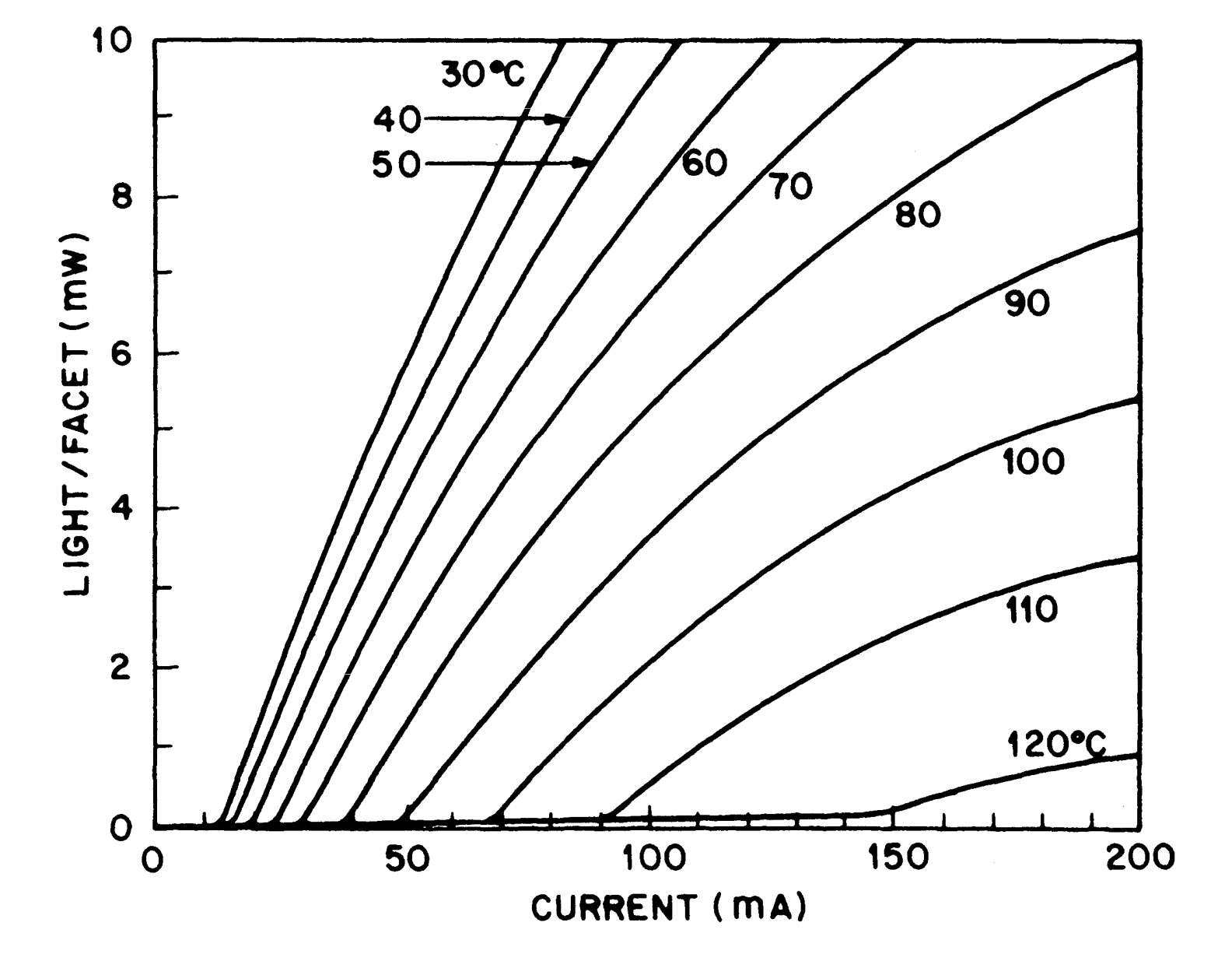Figure 5-28. $$\text{L}-\text{I}$$ characteristics of a 1.3-μm InGaAsP CSBH laser at different temperatures.

Lateral mode control, needed for the kink-free operation up to high powers, can be achieved by controlling the dimensions of the active region. Experimental data for the kink power as a function of the area of the active region is shown in Figure 5-29 for CSBH lasers.

The active area $$A$$ is defined as $$\frac{2}{3}wd$$, where $$w$$ and $$d$$ are the width and the thickness of the crescent-shaped active region.

Figure 5-29 shows that fundamental transverse mode operation can be achieved for lasers with an active area less than $$0.3\text{ μm}^2$$. This result is similar to that shown in Figure 5-23 for EMBH lasers.Figure 5-29.  Measured kink power as a function of active area in CSBH lasers. Data from four different wafers are shown. Arrows represent kink powers greater than 10 mW.

Mesa-Substrate Buried-Heterostructure (MSBH) Laser

Figure 5-30 shows an SEM cross section of an MSBH laser. This device structure can be fabricated using one LPE growth.

The device fabrication involves the following steps.

A mesa 7-8 μm wide and 5 μm deep is etched on a (100)-oriented n-InP substrate. Mesa etching is done using a solution of $$\text{HCl}$$ and $$\text{H}_3\text{PO}_4$$ and a plasma-deposited $$\text{SiO}_2$$ stripe mask oriented parallel to the (011) direction.

After etching, the $$\text{SiO}_2$$ stripe is removed and a type-converted region (p-InP) is created using sealed-ampule $$\text{Cd}$$ diffusion.

Then four epitaxial layers are grown:

• (i) an n-InP buffer layer
• (ii) an undoped InGaAsP ($$\lambda=1.3$$ μm) active layer
• (iv) a p-InGaAsP contact layer.

The wafer is then processed into laser chips using standard fabrication procedures.

MSBH lasers typically exhibit room-temperature threshold currents in the range of 15-30 mA.

The MSBH laser was initially fabricated without the $$\text{Cd}$$-diffused layer. In the absence of a p-type current-blocking layer, these devices have a somewhat higher threshold current compared with that of the EMBH, DCPBH, and CSBH lasers due to a higher leakage current; i.e., the current flowing around the active region is higher in the absence of a current-blocking layer.

The lateral mode control in this laser structure is also achieved by controlling the dimensions of the active region in a manner similar to CSBH lasers.

Other Device Structures

One of the technological issues associated with the fabrication of high-performance index-guided lasers is the control of leakage current.

The device structures described above utilize junctions between different semiconductors for confining the current o the active region.

Many laser structures, some of which are variations of these structures, use semi-insulating layers (e.go., Be- or Fe-doped InP) or highly resistive layers (undoped InP) for current confinement.

Figure 5-31 shows three such structures. They are

• (a) a CSBH laser with an Fe-implanted high-resistivity layer for current confinement
• (b) a vapor-phase mass-transported buried-heterostructure laser employing an undoped InP layer for current confinement
• (c) a mushroom-stripe buried-heterostructure laser with $$\text{SiO}_2$$ layers for current confinement

The main advantage of these structures appears to be the reduction in electrical parasitics because of the absence of reverse-biased junctions. As a result, the device is capable of high-frequency modulation.Figure 5-31.  Schematic illustration of three InGaAsP laser structures: (a)  CSBH laser with an Fe-implanted layer; (b) mass-transported buried-heterostructure laser; and (c) mushroom-stripe laser.

## Leakage Current

In buried-heterostructure lasers of the types shown in Figures 5-18 and 5-19, the confinement of the injected current to the active region is achieved through reverse-biased homojunctions or forward-biased heterojunctions.

The difference between the total current $$I$$ injected into the laser and the current $$I_\text{a}$$ passing through the active region is the leakage current $$I_\text{L}$$, i.e.,

$\tag{5-6-1}I_\text{L}=I-I_\text{a}$

The magnitude of $$I_\text{L}$$ and its variation with $$I$$ depend on the thickness and the carrier concentration of the current-confining layers.

As discussed in the emission characteristics of semiconductor lasers tutorial, a large leakage may lead to a high threshold current and also to sublinearity or power saturation in the light-current characteristics.

Figure 5-32 shows $$\text{L}-\text{I}$$ curves for CSBH lasers with high and low leakage at two different temperatures. The dashed curves represent the slope efficiency ($$\text{d}L/\text{d}I$$ versus $$I$$), which decreases with an increase in the total current for the high-leakage device. Note also that the performance of the high-leakage device degrades severely at high temperatures.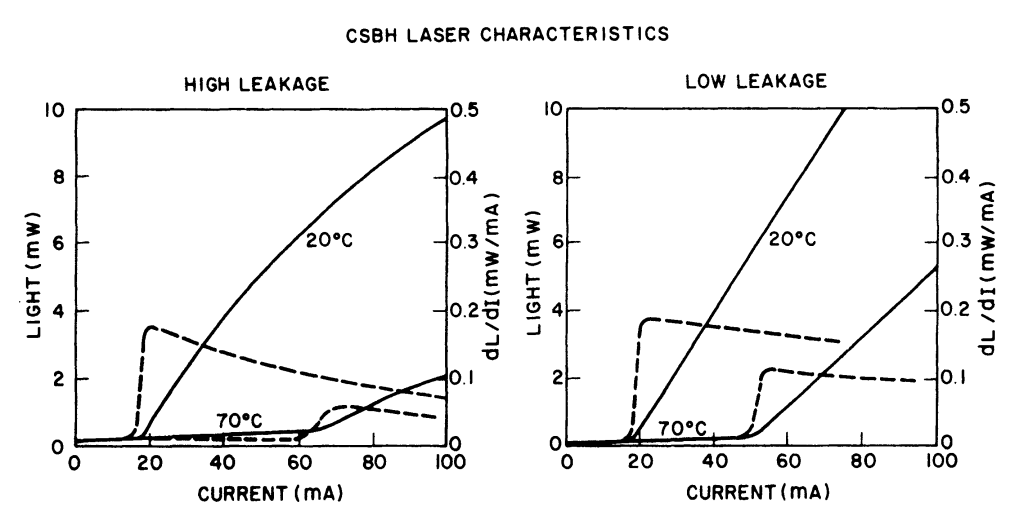Figure 5-32.  $$\text{L}-\text{I}$$ characteristics at two different temperatures for two CSBH lasers with high and low leakage currents. The dashed curves show the slope efficiency.

Before going into details of leakage paths in various laser structures, we first consider physically how a forward-biased junction can confine the current at low injection and become "leaky" at high injection.

For the purpose of illustration, we consider an EMBH type of laser structure. Figure 5-33(a) shows two current paths for $$I_\text{L}$$ and $$I_\text{a}$$ and the corresponding equivalent electrical circuit model is shown in Figure 5-33(b).

The laser and the InP homojunction are represented by forward-biased diodes in the equivalent circuit. $$R_\text{s}$$ is a resistor in the laser diode path and the $$R_2$$ is a resistor in the path of the InP homojunction diode.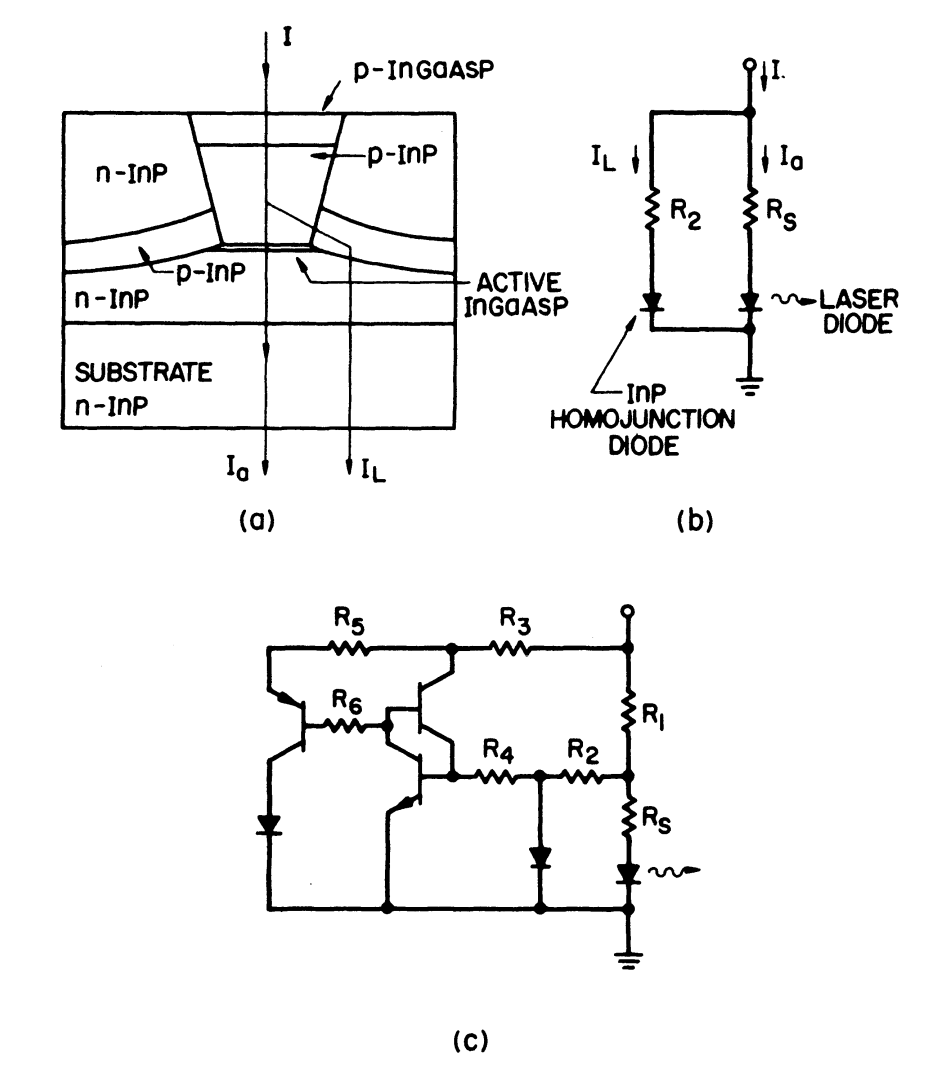Figure 5-33.  (a) Schematic cross section of an EMBH laser. (b) Simplified electrical equivalent circuit of a laser diode in parallel with an InP homojunction diode. (c) Electrical equivalent circuit of an EMBH laser.

Figure 5-34 shows schematically the current-voltage ($$\text{I}-\text{V}$$) characteristics of the laser diode with the series resistance $$R_\text{s}$$. The dashed line is the $$\text{I}-\text{V}$$ characteristic of the homojunction-leakage diode.

Figure 5-34 shows that as the external voltage is increased, most of the current initially passes through the active region, until the voltage reaches the turn-on voltage of the leakage diode. With a further increase in voltage, the fraction of leakage current increases, and at high current, the majority of the injected current flows through the leakage junction.Figure 5-34.  $$\text{I}-\text{V}$$ characteristics of the laser diode path (solid curve) and the homojunction diode path (dashed curve). The two paths are shown in Figure 5-33(b), as $$I_\text{a}$$ and $$I_\text{L}$$, respectively.

We now discuss the leakage current paths in an EMBH laser. The laser structure and its equivalent-electrical-circuit model are shown in Figures 5-33(a) and 5-33(c).

For the purpose of discussion, the leakage current paths are identified by a major circuit element in each branch, e.g., the diode (p-n forward-biased InP homojunction), the transistor (n-p-n InP), and the thyristor (p-n-p-n InP). Various layers participating in the three leakage current paths are described below (refer to Figure 5-20 for the terminology).

For the diode leakage path, the forward-biased p-InP blocking layer and the n-InP buffer layer form the diode. The effective saturation parameter for this diode is determined by current spreading in the p-InP layer. Current spreading is less for thinner p-InP layers; hence the diode leakage is expected to be smaller for a thin p-InP layer.

The n-p-n transistor in the transistor leakage path consists of the n-type buffer layer (emitter), the p-InP blocking layer (base), and the n-InP top regrown layer (collector). Increasing the width of the p-InP blocking layer increases the base width of the transistor, which reduces the current gain of the transistor and hence the leakage through the transistor path.

The various layers corresponding to the p-n-p-n thyristor path are the p-InP cladding layer, the n-InP top regrown layer, the p-InP blocking layer, and the n-InP buffer layer. At low currents the leakage through this path is negligible because the thyristor is off. However, at high currents when the thyristor is turned on, the leakage current through this path may be significant.

The magnitude of the leakage current through each of the leakage paths shown in Figure 5-33(c) can be calculated by analyzing the equivalent electrical circuit.

The results of the calculation depend strongly on the saturation parameters of the various circuit elements and on the ideality factor of the diode. These quantities are determined by the quality of the interface between layers, in addition to layer thicknesses and the doping levels.

The interface quality is determined by the details of device fabrication such as types of etching agents used, growth temperatures, and conditions prior to regrowth that affect the thermal decomposition of exposed surfaces.

These factors complicate the comparison of the experimental results with the calculated light-current characteristic obtained with the electrical-equivalent-circuit model.

Figure 5-35 shows representative results. The dashed curve is the leakage current, principally through the thyristor path, and the solid curve is the light-current characteristic. The calculated light output per facet is obtained using an external differential quantum efficiency of 0.2 mW/mA per facet.

Due to the increase in the leakage current, the output power saturates at high currents. Under CW operation there is an additional heating effect that can "roll over" the light-current characteristics; i.e., the light output can decrease with increasing current.Figure 5-35.  Calculated $$\text{L}-\text{I}$$ characteristic of an EMBH laser after including the current leakage paths modeled by the circuit shown in Figure 5-33(c). The dashed curve shows the leakage current.

The next tutorial discusses about laser arrays